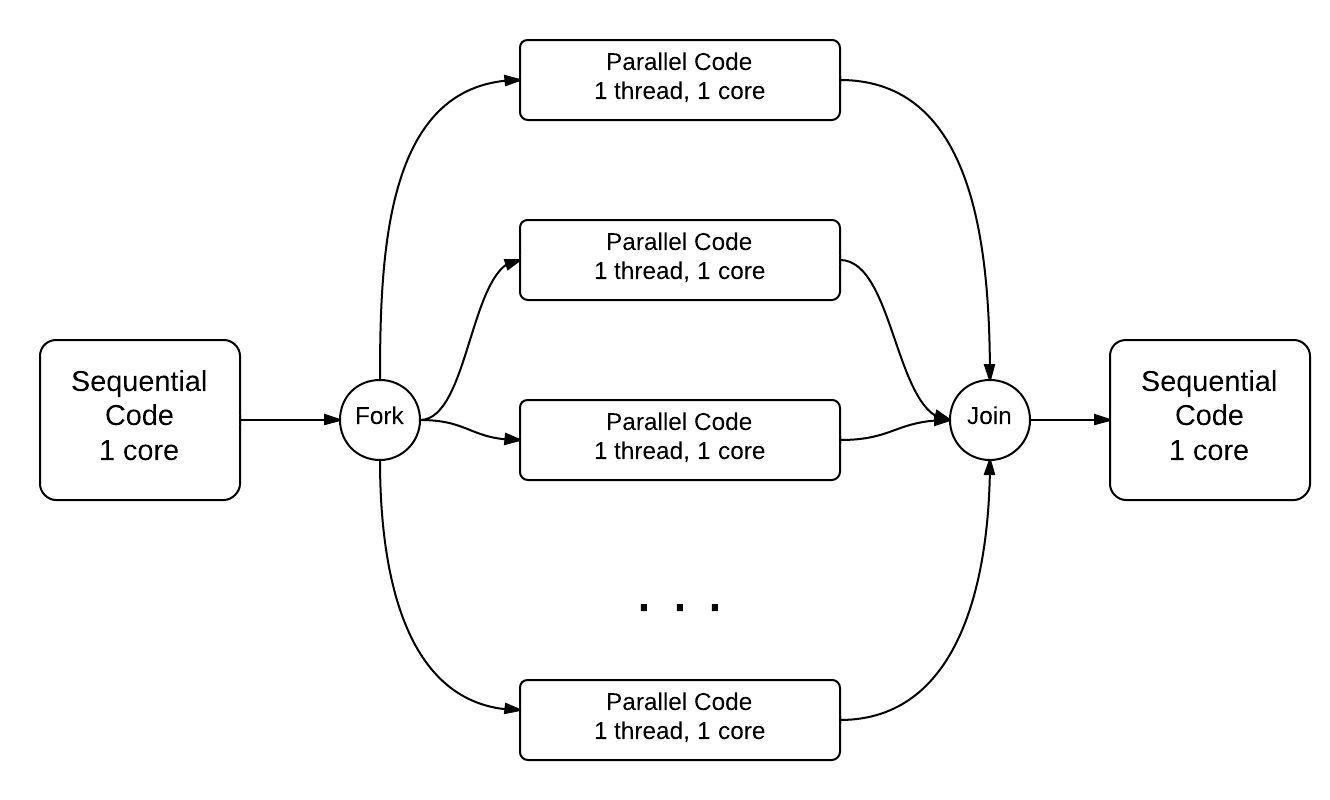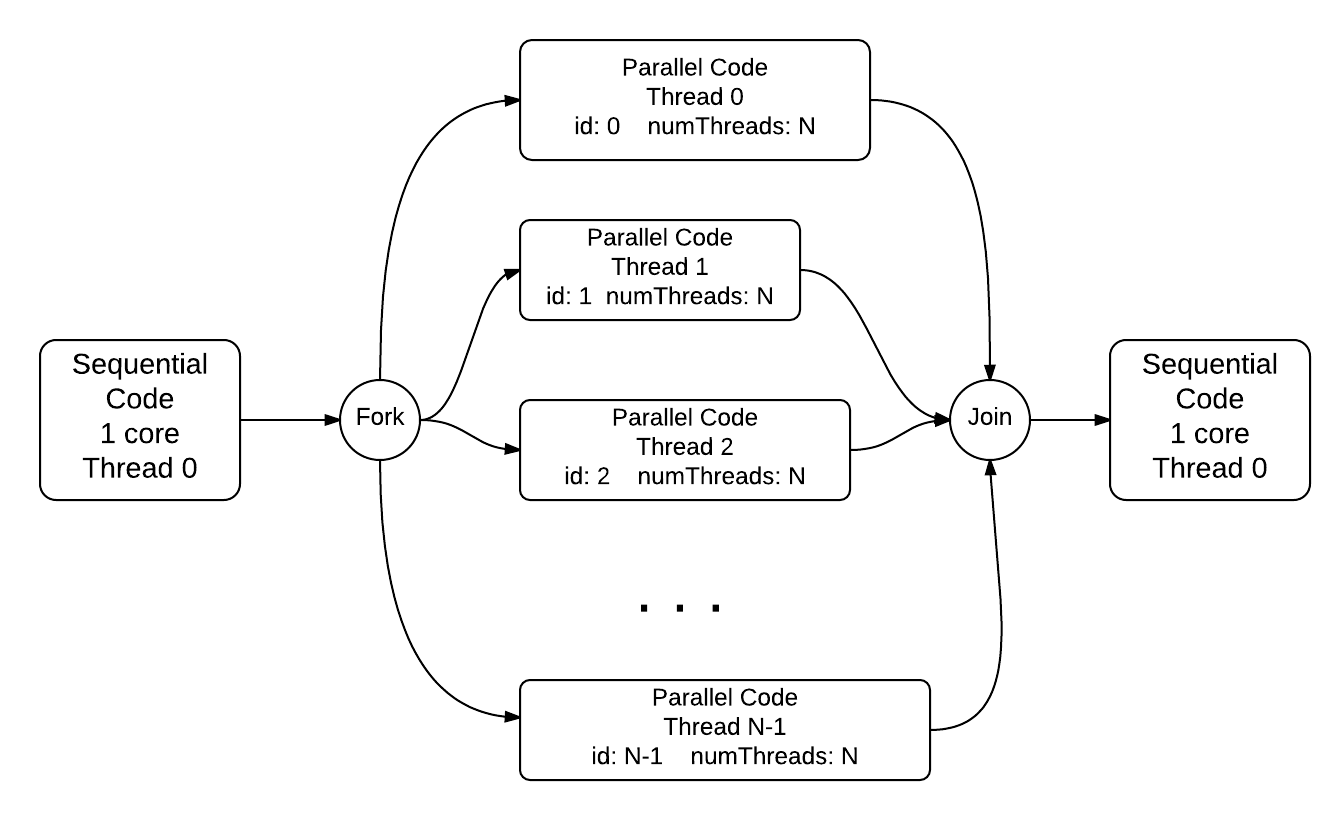# 1.1 First Steps - Programming Shared Memory¶

In this book, we use the Open Multi-Processing (OpenMP) library to demonstrate how to program shared memory systems. While OpenMP is just one of many options for programming shared memory systems, we choose OpenMP due to its pervasiveness and relative ease of use. OpenMP employs a series of compiler directives to enable users to incrementally add parallelism to their programs and is natively supported by GCC.

## 1.1.1 The Fork-Join Pattern¶

As a means of introducing OpenMP, we begin by introducing the fork-join pattern for parallel programming.In fork-join, the main thread “forks” (or creates) a series of threads that each perform a separate task in parallel. When each thread terminates, their executions “join” (or merge) together back into the main thread.

Consider the following serial program. What is its output?

The above code simply prints out the strings Before, During and After in order.

Now uncomment the omp parallel pragma on line 8 and re-run the program.

The omp parallel pragma on line 8, when uncommented, tells the compiler to fork a set of threads to execute the next line of code (later you will see how this is done for a block of code). Next, the omp parallel pragma creates a team of threads and directs each thread to run the line printf(\nDuring...) in parallel.

Thus, the string During is printed out a number of times that correspond to the number cores on the system (in this case, 4). Note that in OpenMP the join is implicit and does not require a pragma directive.

## 1.1.2 The SPMD Pattern¶

A common use of the fork-join pattern is to have each thread run the same block of code on different components of data. This pattern is known as single program multiple data or the SPMD pattern. Let’s try running a new code snippet:

When the omp parallel pragma executes, it assigns each thread a unique id (from 0 to n-1 for n threads). A programmer can access this unique id by calling the omp_get_thread_num() function. Likewise, OpenMP provides the omp_get_num_threads() function to provide the programmer the ability to see the total number of threads. On a single threaded program (like the one shown above), there is 1 total thread, with a thread id of 0.

Consider what will happen when the pragma above is uncommented (recall that there are 4 total cores on the system). What do you think the output would be?

Let’s now run a version of the program with the omp parallel pragma uncommented:

Running this program reveals two things. First, since there are 4 total cores on the system, the omp parallel pragma generates a team of 4 threads, assigning each a unique id from 0 to 3. Each thread then runs the code in the scope of the pragma (denoted by curly braces). The process can be visualized as follows:The code in main up until line 6 is run in one thread on one core; the forking of separate threads to run the code between lines 7 and 12 is shown in the middle of the diagram. The final last couple of lines of code are run back in the single thread 0 after all the threads have completed and join back to the main thread.

Re-running the program multiple times illustrates an important point about threaded programs: the ordering of execution of statements between threads is not guaranteed. In fact, the order in which any set of threads execute is not guaranteed, and is determined by the operating system. This situation illustrates the concept of non-determinism, where an algorithm or program can have different outputs over multiple runs. While all parallel algorithms have inherent non-deterministic properties, experienced programmers can leverage the non-deterministic execution to their advantage (e.g. run the code on multiple cores) and still get correct output. We will study several such examples in the coming sections.

## 1.1.3 A Larger Example - Filling an Array¶

The fork-join and SPMD patterns are some of the most widely used for programming shared memory systems. In general, the fork-join pattern is used for task parallelism, or when a team of threads receive a component of a larger problem and work together to come up with a solution. The SPMD pattern is commonly used for data parallelism where a team of threads run the same program on different components of data or memory. In this scenario, each thread does the exact same task – the only difference is that each thread is operating on a different unit of data or memory.

As an example, consider the process of filling an array of size n with elements from 1 .. n -1:

The following snippet of C code is a serial implementation that populates an array with 20 million elements:

Let’s consider how we would parallelize a program like this. One way is to assign each thread a different segment of the array, and have each thread populate its own component of the array. The following video illustrates how 4 threads would populate an array (each thread is assigned a different color):

The notions of “task parallelism” and “data parallelism” are two extremes on a spectrum. Most parallel programs fall somewhere along the spectrum. For now, it is sufficient to recognize that both fork-join and SPMD are valid ways to assign work to threads.

## 1.1.3 For-Loop Pragmas¶

Before we parallelize the populate array program, we need to introduce two new pragmas. The first is the omp for pragma. This pragma parallelizes the iterations of a for loop by assigning each thread a chunk of iterations of the loop. The following code snippet illustrates how to use the omp for pragma to parallelize the populate array program:

Notice that in the body of the above program that there is nothing between the omp parallel and the omp for pragmas. This is fairly common, as sometimes the key piece of code to be parallelized is just a for loop. To simplify the process for programmers, OpenMP provides the omp parallel for pragma, which literally combines the functionality of the omp parallel and the omp for pragmas into one line of code.

The following program illustrates this new pragma in action:

Notice how much shorter and simpler this code is. However, the omp parallel for isn’t always appropriate for all cases, as we will see in the next section.